# Guide To Fractions in 10 Simple Facts

Mathematicians love fractions while the rest of the world hates them. I’m not sure what kind of traumatic events y’all went through in your childhood, but I’m sorry they were so painful.

In TEN FACTS I will break down everything you need to know about fractions as clearly as possible.

Yes, one lesson, ten essential ideas, less than ten minutes of reading.

# Fraction = number of parts / total parts

Every fraction has a numerator that equals the number of parts we have and a denominator equaling the total number of parts in a whole.

Just like cake you could have 2 small pieces or 1 piece twice as big and it’s the same amount. Hence a lot of fractions are equivalent, like 2/5 and 4/10.

# Any Number Can Be Written As a Fraction

Write any whole number over 1 to make it a fraction since the total number of parts in any undivided whole is one.

# Multiply Fractions Straight Across

Multiplying fractions is easy, just multiply straight across.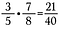3 x 7 = 21 and 5 x 8 = 40

# Multiply By Any Form of One Anytime

The number 1 is called the multiplicative identity because we can multiply it by any number and the number remains the same. This is important for fractions because often times we need to alter the appearance of a fraction without actually changing its value.

For example, I can change 1/3 into the equivalent fraction 3/9 by multiplying by 3/3.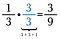Multiplying by 1 in the form of 3/3 turns 1/3 into the equivalent fraction 3/9

# Add and Subtract Equal Sized Parts

When adding and subtracting fractions the denominators must be the same. That makes sense. If we wish to combine or take away parts we must be talking about the same sized parts, otherwise it would get confusing.

So what do you do if your fractions do not have the same sizes?

Multiply by a form of one to change the denominators into a common size. Essentially, we’re dividing the fractions into smaller sized pieces until they’re the same size. This is called finding a common denominator.

Truthfully, any common denominator will do, but people prefer to find the smallest one. In this case the smallest number both 7 and 3 go into without a remainder is 21. So multiply the first fraction by 3/3 and the second by 7/7.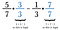Multiply by forms of 1 to make a common denominator of 21.

If you can’t think of the least common denominator, you can always multiply each fraction by the opposite denomination. Sometimes, as in this case, that turns out to be the least common denominator. If it isn’t, just reduce your answer at the end.

Once the denominators are matching, subtract the numerators to get 8/21.

This works as one would expect. Pictorially, begin with 15 pieces of 21 total.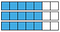Notice I have 5/7’s replicated 3 times, this directly relates to multiplying 5/7 by 3/3 to obtain 15/21.

Remove the coloring from 7 of the 15 blue blocks.

Which leaves 8/21 as expected.

# Change Out Mixed Numbers First

A mixed number is the combination of a whole number and a fraction.

Mixed numbers don’t play well with other fractions. It’s a good idea to convert them into improper fractions first.

Note: An improper fraction is a fraction whose numerator is larger than its denominator therefore having a value greater than one.

# Converting a Mixed Number is Really Addition

To convert 2 and 4/5 into an improper fraction add 2 + 4/5.

Step 1: Begin by rewriting 2 as 2/1.

Step 2: Multiply 2/1 by 5/5 to make an equivalent fraction of 10/5 which has the desired common denominator of 5.

Step 3: Add 10/5 + 4/5.

Our result is the equivalent improper fraction 14/5.

To convert back to a mixed number perform the division. For example, 5 goes into 14 two times (since 5 x 2 = 10) with 4 pieces left over.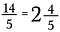Equivalent fractions in improper form (left) and mixed number form (right)

# Compare Fractions Using The Cross Product

Suppose we wanted to determine which is bigger: 5/12 or 6/13.

Step one: Multiply a diagonal and write the product above the numerator.

Step two: Multiply the other diagonal and write its product above its numerator.

Step three: Compare the products. The side with the larger product is the larger fraction. So in this case, 5/12 is less than 6/13.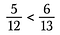Note: the greater than/less than symbol always opens toward the larger value.

We can determine if fractions are equal using cross products too.

The cross product of 3/7 and 12/28 are both 84, therefore 3/7 = 12/28.

# Cancel Anything That Divides to One

The best thing about fractions is that you can find plenty of opportunities to cancel. Which makes them quick and easy to manage.

Suppose I have the fraction 8/10. Both 8 and 10 can be rewritten with 2 as a factor.

Because 2/2 = 1, I can cancel out the 2’s leaving 4/5 as the reduced fraction.

Utilize this strategy to make multiplying fractions easier as well.

Begin by rewriting each numbers in factors.

Cancel any number pairs that divide to 1. For instance, 5/5 = 1.

I have another pair of 5’s as well as a pair of 3’s that divide to 1 as well.

Oops! I could have rewritten 6 as 2 x 3 and cancelled a pair of 2’s. It’s okay if you miss a factor, just keep going until you get them all.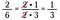Note: I rewrote 2 as 2 x 1 so that when I cancel out the 2’s I am left with a one in the numerator.

Had multiplied 15/25 times 10/18 directly it would have been a lot of arithmetic, using canceling I pre-reduce the fractions and make it simpler to multiply.

# Use Multiplication to Divide Fractions

The concept of dividing fractions is easy with simple examples such as:

There are two halves in a whole, therefore there are 10 halves in 5 wholes.

But the concept gets tricky with more complicated fractions.

To solve this problem we’ll utilize two facts:

1. We can multiply by any form of one (i.e anything over itself)
2. Multiplying by the reciprocal of 3/2, which is 2/3, results in 1 via canceling

Step one: Begin by multiplying by the reciprocal over itself.

Now we have two smaller problems to solve (blue and green).

Step two: Cancel out anything that divides to 1 in the bottom (green) fraction. This should always result in 1.

Now we are left with the top problem to solve.

Step three: Use canceling to pre-reduce the fraction. After making these reductions, multiply across to obtain 4/3.

## Shortcut

This is the long hand mechanics of flip and multiply.

We can skip multiplying by the reciprocal on bottom since it always cancels to 1. Therefore all you need to do is multiply the numerator by the reciprocal of the denominator.

# Bonus: Theory, in case you’re interested…

## Why does the cross product trick work?

Great question! To generalize, make two fractions using the letters a, b, c, and d to represent four different numbers.

Multiply both fractions by b•d (this will enable us to cancel out the denominators).

Now cancel the b’s on the left and the d’s on the right since they divide to 1. We no longer have fractions, just the products d•a and c•b.

Look back at the original fractions. These are the same products as if we had multiplied the diagonals. Therefore, the shortcut is to compare the cross product.

## ❤ STAY CONNECTED ❤

Stay up-to-date with everything Math Hacks is up to!

Written by

Written by

## Brett Berry

#### Check out my YouTube channel “Math Hacks” for hands-on math tutorials and lots of math love ♥️## Math Hacks

#### Tutorials with a fresh perspective.

Welcome to a place where words matter. On Medium, smart voices and original ideas take center stage - with no ads in sight. Watch
Follow all the topics you care about, and we’ll deliver the best stories for you to your homepage and inbox. Explore
Get unlimited access to the best stories on Medium — and support writers while you’re at it. Just \$5/month. Upgrade